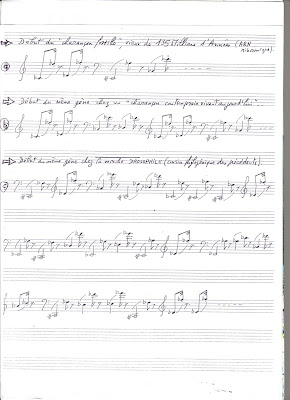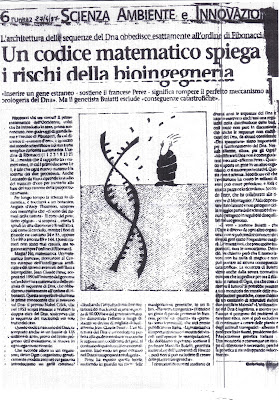## more on SCRIBD... and in the book CODEX BIOGENESIS...

============================

https://sites.google.com/site/codexbiogenesis/home

http://www.resurgence.be/90-codex-biogenesis-9782874340444.html

read the book on AMAZON here...

...a research starting then published 20 years ago...please, clic here...

October 2013: a new publication demonstrating why human genome is a WHOLE...

## jeudi 24 janvier 2008

### 1997: The Mendeleiev Periodic Table Predictive Equation

se also in Belgrade University web site http://www.mi.sanu.ac.rs/vismath/perez2009/perez.pdf:## of Mendeleiev’s Periodic Table

“Are there Numbers in the Nature?” Let us take the example of the famous table of Mendeleev, no one never had the idea to seek a possible mathematical law which would organize the information and the structure of "the most heterogeneous table of Science".

Jean-claude perez discovered this law: the equation of the periodic table of Mendeleev. Here is a short summary: Pionnering in research of numbers in DNA, this researcher was questionning about possible DIFFERENCIATION between life based Elements (carbon oxygen etc) and all others (aluminium, copper etc). In fact, he discovered the exact contrary: a law of UNIFICATION of all Elements of Nature... Then he discovered a very simple equation which generates and predicts the structure of the table of Mendeleev.

-This equation predicts the number of elements of any layer of period "p" in the table according to the only value of this period "p". Beyond this mathematical modeling of the periodic table of the Elements,

-This equation underlines, in its formulation, the " trace" of the 4 fundamental quantum Numbers.

-This modeling predicts the structure of the hypothetical extensions of the table of Mendeleev towards possible Eléments (real) unknown which would be located beyond the last known radioactive Elements.

-This modeling also makes it possible to imagine an infinity of other Elements (virtual) which one could however predict positioning towards the low layers of the table, like their quantum properties.

To summarize, IF:

`-c(p) a horizontal layer of elements of the table of Mendeleev, `
`-"p" the period associated with this c(p) layer such as p = [ 1 2 3 4 5 6 7 … ],`
`-Int(v) the whole part of the numerical value "v". exp: if v=2.35,then Int(2.35)=2.`

THEN: one obtains c(p), the number of elements contained in the c(p) layer of order p, by applying the formula:

`    c(p) = 2 [Int ((p+2) /2 )]*2`

Examples :

`If p=1 then c(1)=2  `
`If p=2 then c(2)=8    If p=3  then c(3)=8`
`If p=4 then c(4)=18   If p=5  then c(5)=18`
`If p=6 then c(6)=32   If p=7  then c(7)=32`
`If p=8 then c(8)=50   If p=9  then c(9)=50`
`…/…                   If p=16 then c(16)=162`

To conclude : -1- The periodic table of the Elements is modélisable. It is structured by a numerical structure of whole numbers. -2- This structure is deterministic and predictive, then, for any period p, it can be calculated by applying "the Perez's generic equation of Mendeleev". -3- The generic equation is completely controled by the four quantum Numbers. -4-This generic equation makes it possible to check the regularity of the common table of Mendeleev, but it can also "predict" and anticipate the existence of hypothetical Eléments now unknown, of which it makes it possible to determine the quantum properties, then electronic and chemical hypothetical properties.

Please see a detailed paper zooming text image at the top of this paragraph...

## mercredi 23 janvier 2008

### 1994: The First Music of Genes2 examples of the 1st MUSIC of GENES:
Evolution from a fossil charançon towards drosophile
and
music of the KIRAS ONNCOGENE...Please clic on images to zoom...

Facing this image of dynamics of DNA supracode resonances, I had immediately the intuition to show this pattern on another support: MUSIC...

Then, to explore both aesthetics dimension of this discovery, Perez published in 1994 an audio CD entitled

So, now, if you CLIC on the following text...

"The First Music Of Genes" (Introduction, track 1)

Then you enter in the AUDIO CD Introduction track of - SACEM GEN0694 - (a subset of supracode for the oncogene KIRAS involved in cancers)...

Now a dedicaced GOOGLE WEB SITE is available here... its name: TheFirstMusicOfGenes

## jeudi 17 janvier 2008

### 1997: 2 books: "PLANèTE TRANSGENIQUE" on GMO risk and « l’ADN decrypté” (1997) – DNA decypheredSOME WORK ON GMO RISK...Relative proportions of TCAG nucleotides within DNA of genes are structured by Fibonacci/Lucas numbers: there is the "SUPRA CODE OF DNA" discovered by jean-claude Perez in 1991 and fully detailled in this book in 1997..."We suggested with Jean-Claude Perez working on experiments which we had already made. He quickly fell on an important area within the HIV1 virus which we had spent four years to find".

Professor Jean-claude CHERMANN,
Co-discoverer of the AIDS virus - INSERM - Marseille -
In "Objectif Mediterranée" French economic magazine
May 1993 (1).

" The principle of Jean-Claude Perez's discovery appeals to me. Indeed, it is quite possible, that, beyond the sequences, there is a kind of "supra-code" of the DNA .
That could particularly explain these sequences within the DNA which do not code, which are not used for the synthesis of the proteins and of which one says that they are not used for nothing…
"

Professor Luc MONTAGNIER, the discoverer of HIV virus
New-york University -
In " Sciences et Avenir ",
" la musique des gènes " April 1995 (2)

DNA SUPRACODE SUMMARY:

We have discovered in 1990 then published from 1991 a mathematical law controlling the self-organization of T C A G nucleotides within DNA.
The sequential sets of DNA nucleotides are organized in long-range structures entitled "RESONANCES". A resonance is a particular proportion doing a partition of DNA according with "Fibonacci's Numbers series" (1 2 3 5 8 13 21 34 55 89 144 etc…). There are the same proportions which those controlling morphology of Natural organisms as Nautilus, apple-pines etc…
Example: consider 144 contiguous nucleotides of DNA resulting from 55 bases T and 89 bases A C G, then, this (55-89-144) proportion is called a "Resonance".
We find thousands resonance within DNA : example, in the HIV virus, long of about 9000 bases, there are thousands resonance (50000), and particularly, very long 6000-resonances overlapping 2/3 of this genomic DNA.
In the mitochondria DNA which is a circular genome of 16500 bases long, we have discovered transgenic possible combinations providing emergence of FIVE MILLION bases length hyper-resonance (see part II of this present paper).
We conclude that NATURAL GENES and DNA are characterized by high-level resonance structures.
In other hand, "entropy" and a poor low-level of resonance generally characterize ARTIFICIAL DNA transgenic sequences.
The physical nature of this structure is located at the DNA STABILITY level.
Then there are many ways of applications and perspectives by example in "antisens" technology, principally in the new promising domain of RNA interference. etc…
This discovery, done in 1990 by Jean-Claude Perez, then researcher in IBM, lengthily was tested and validated since specifically with GeNum start-up Lab., in particular on the HIV genome of the AIDS with Professors Chermann, then Montagnier, both the first discoverers of the AIDS virus.

COMPUTING “DNA Fibonacci/Lucas RESONANCES”

There is this Fibonacci's proportion which is the key of my discovery of the Supra-code of DNA. It is stated as follows :

Let us consider, anywhere in a sequence of DNA, a contig of bases TCAG of which the length is a number of Fibonacci : for example 144 bases.

If this section is subdivided, for the example treated here, in 55 bases T and 89 bases A or C or G... We will thus say that this unit constitutes a " resonance ".

" A resonance is thus a proportion between 3 successive numbers of Fibonacci. "

All combinative of other combinations is possible:

C versus AGT, A versus TCG, or G versus TCA by example.

One will insist on the fact that the relative positions of the bases inside this section are indifferent. It is before a a whole question of RATIO and relative PROPORTION.

The SUPRA-CODE of the DNA is thus the whole of resonances which one finds, thus, by the systematic exploration of sections of DNA contigs.

One is astonished by the richness and the complexity which form this network of resonances.
One very finds of course as many resonances in the 95% of "junk DNA" than in the 5% of the DNA known as "functional and useful" and "coding for proteins".

In order to give an idea of this "architecture", let us consider the complete genome HIV of the AIDS viruses, it comprises only 9000 bases and practically not "junk DNA":

One discovers there more than 50000 resonances significant lengths.

Among them, more than 10000 resonances are higher than 300 bases and more than one thousand of resonances covers more than 2000 bases.

Several hundreds of resonances "cover" more than 6000 bases, then, TWO THIRDS of the WHOLE GENOME !